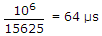# Electronics and Communication Engineering - Communication Systems

1.

In a satellite system circular polarization is to be obtained. The antenna used is

 A. parabolic B. horn C. log periodic D. helical

Explanation:

Helical antenna produces a circularly polarized radiation if its two components are at right angles and are equal. It is very commonly used in radio telemetry.

2.

A horizontal output stage is cutoff for retrace and 40% of trace. If time for each horizontal line is 64 μs and retrace time is 12 μs, the transistor is conducting for about

 A. 64 μs B. 6.4 μs C. 31 μs D. 3.1 μs

Explanation:

Total time for each horizontal line =.

Time for trace = 64 - 12 = 52 μs.

Time for which transistor is not conducting = 12 + 0.4 x 52 = 32.8 ms.

Time for which transistor is conducting = 64 - 32.8 = 31 μs.

3.

A TV remote control works on the principle of

 A. pulse code modulated ultra violet light B. pulse code modulated infrared light C. demodulation D. either (a) or (b)

Explanation:

Infrared light is used in TV remote control.

4.

As the magnitude of reverse bias is increased, the capacitance of varactor diode

 A. increases B. decreases C. first increase, then decreases D. first decreases then increases

Explanation:

When magnitude of reverse bias is increased, thickness of depletion layer increases.

Capacitance is inversely proportional to thickness.

5.

 A. variable sensitivity B. squelch C. double conversion D. variable selectivity